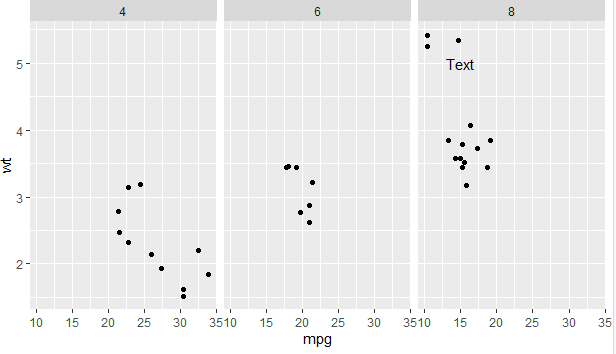2 views

I want to annotate some text on the last facet of the plot with the following code:

library(ggplot2)

p <- ggplot(mtcars, aes(mpg, wt)) + geom_point()

p <- p + facet_grid(. ~ cyl)

p <- p + annotate("text", label = "Test", size = 4, x = 15, y = 5)

print(p)But this code annotates the text on every facet. I would highly appreciate if you guide me on how to get the annotated text on only one facet. Thanks in advance.

by

To annotate text on the last facet, add the following to your code:

text_ann <- data.frame(mpg = 15,wt = 5,lab = "Text",

cyl = factor(8,levels = c("4","6","8")))

p + geom_text(data = ann_text,label = "Text")

i.e.,

library(ggplot2)

ggplot(mtcars, aes(mpg, wt)) + geom_point()

p + facet_grid(. ~ cyl)

ann_text <- data.frame(mpg = 15,wt = 5,lab = "Text",

cyl = factor(8,levels = c("4","6","8")))

p + geom_text(data = ann_text,label = "Text")

Output: